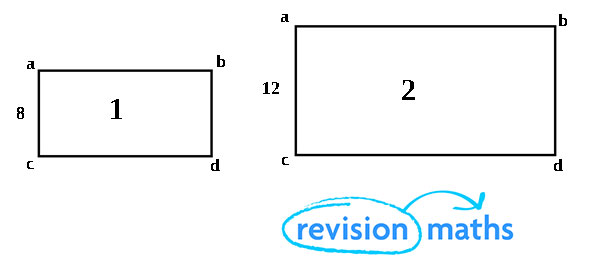## Scale Factors

Scale Factors, this GCSE Maths section of Revision Maths explains scale factors and how to calculate them.

The video below provides an overview to calculating scale factors.

Scale Factors

A scale factor is a number which is used as a multiplier when scaling.

Scale factors can be used to scale objects in 1, 2 or 3 dimensions. They can be written as either ratios, decimals, fractions or percentages.

Linear Scale Factor

Using linear scale factor a shape can be transformed into another similar shape by changing the size of all its dimensions (either enlargement or reduction) by using a scale factor.

To enlarge or reduce any shape you must begin by working out the scale factor, this is calculated by using the following formula:

For an enlargement = large number ÷ small number

For a reduction = small number ÷ large numberIn the example above we are looking for the length of the bigger rectangle 2. This is therefore an enlargement so we use the following formula 12 ÷ 8 = 1.5.

So we therefore know that rectangle 2 is 1.5 times bigger than rectangle 1.